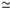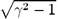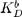# Abstract

A new regime of runaway discharges has been observed in the TCABR tokamak. One of the most distinctive features of this regime is the effect of plasma detachment from the limiter. This experimental fact can only be explained by the volume recombination, which requires a low-temperature plasma. The analysis of the energy and particle balance in the system plasma-relativistic runaway beam in TCABR, which takes into account only the collisional mechanism of the heat transfer from runaways to thermal electrons, predicts electron temperatures Te = 0.1 - 2 eV; the temperature decreases with the neutral density increase. The recombination process with the rate constant around 10-16 m³/s is required for the explanation of plasma density behavior in the experiment. At present, it is difficult to conclude about the mechanism of recombination. More reliable and detailed experimental data, mainly about the plasma temperature, are necessary.

Plasma Recombination in Runaway Discharges in Tokamak TCABR

T.K. Soboleva1, R.M.O. Galvão2, S.I. Krasheninnikov3,

Yu.K. Kuznetsov2, and I.C. Nascimento2

1Instituto de Ciencias Nucleares, Universidad Nacional Autonomia de Mexico D.F., Mexico

2 Instituto de Física, Universidade de São Paulo,

C.P. 66318, 05315-970, São Paulo, Brasil

3 University of California, San Diego, USA

A new regime of runaway discharges has been observed in the TCABR tokamak. One of the most distinctive features of this regime is the effect of plasma detachment from the limiter. This experimental fact can only be explained by the volume recombination, which requires a low-temperature plasma. The analysis of the energy and particle balance in the system plasma-relativistic runaway beam in TCABR, which takes into account only the collisional mechanism of the heat transfer from runaways to thermal electrons, predicts electron temperatures Te = 0.1 - 2 eV; the temperature decreases with the neutral density increase. The recombination process with the rate constant around 10-16 m3/s is required for the explanation of plasma density behavior in the experiment. At present, it is difficult to conclude about the mechanism of recombination. More reliable and detailed experimental data, mainly about the plasma temperature, are necessary.

I Introduction

A new regime of runaway discharges has been observed in TCABR tokamak , with major plasma radius R = 0.615 m, minor radius of the limiter a = 0.18 m, toroidal magnetic field B = 1.1 T. One of the most distinctive features of this regime, compared to conventional runaway discharges in tokamaks , is the effect of plasma detachment from the limiter. This experimental fact can only be explained by a the volume recombination, which requires a low-temperature plasma. The present work is regarded to the question on how the existence of a recombinative plasma agrees with the energy and particle balance in the presence of an intensive relativistic beam of runaway electrons. The main experimental data related to this topic are presented in Section II. The analysis of energy and particle balance is performed in Section III. The conclusion is given in Section IV.

II Experimental data

A clear indication of the recombinative plasma in the runaway discharges observed in TCABR is the effect of plasma detachment from the limiter. The plasma density in the scrape-off layer (SOL), measured by a Langmuir probe, starts to decrease at the start-up phase and vanishes at the stationary phase (see Fig. 1). At the same time, the line density measurements by the interferometer along different vertical chords indicate a shrinkage of the plasma column. Indeed, the plasma column is shifted outward, due to the high kinetic energy of runaways, and its minor radius is estimated from these measurements to be in the range 0.09 m £ ap£ 0.14 m. These experimental facts can be explained only by volume recombination in a low-temperature plasma. Such plasma exists in the runaway discharges due to the low heating power. Simple estimations indicate that resistive fraction of the plasma current and thus the ohmic heating power are negligible small at low electron temperatures Te < 5 eV. The power transferred collisionally to the plasma from the relativistic low-density runaway beam is also rather small. The low-temperature recombinative plasma detached from the limiter is kept in equilibrium by the runaway current.

Other distinctive feature or this regime is the relaxation instability with strong spikes in Ha emission correlated with sawtooth relaxation of the line density (see Figs 1 and 2). Plasma-beam instabilities can provide an additional plasma heating channel. We conclude from the experimental data that plasma heating occurs in short pulses. Expanding a single sawtooth, we find that the characteristic time of density increase in the case shown in Fig.2 is about of 10 ms. This can be explained by a temperature increase to Te» 15 eV, taking into account that the neutral density in this case is around 1019 m-3. Spikes in Ha, electron cyclotron and X-ray emissions and negative voltage spikes, which we considered as ones related to the instability process, occur in time intervals of tens microseconds. Then it seems that the instability is quenched, the plasma temperature drops and the density decays due to recombination.

Experiments with gas puffing confirm the model of low-temperature plasma and give an additional information about this regime. The runaway discharge is much more stable with respect to the gas puffing as compared to the normal one. The main effects observed under increase of the neutral density, up to 5 × 1019 molecules/m3, are the increase of the frequency of the spikes in all signals, increase of the rate of density decay in the relaxation process, and decrease in the runaway current and background plasma density.

Currently, we have no direct measurements of the plasma temperature, which are difficult due to the effect of runaway beam. Estimations of the electron temperature in the RAD can be obtained from the decay of positive voltage spikes . This method gives electron temperatures 0.2 - 1.5 eV .

The average runaway energy is deduced from equilibrium effects. The value of the beta poloidal is determined from magnetic diagnostics. Then the average energy of runaways is estimated from the value of beta poloidal using the formula 

where IA (kA)17is the Alfvén current and g is the relativistic factor. In our case, Ib

It. For the discharge presented in Fig. 1, we obtain, at the quasi stationary phase, g » 9, which corresponds to kinetic energy of 4 MeV.

III Energy and particle balance calculations

The plasma is transparent to neutrals in our conditions. For simplicity, let us consider zero-dimensional transport model. Energy balance equations for thermal electrons and ions are

where

Q are heat fluxes caused by ohmic heating (

Q

oh), runaway-thermal electrons (

Q

b), electron-neutral (

Q

en), electron-ion (

Q

ei), and ion-neutral (

Q

in) collisions.

Q

b has the form

where Coulomb logarithm is approximately lnL

b = 20. We assume

n

e=

n

i, i.e. the effective ion charge

Z

i = 1. For relativistic runaway beam, the runaway density is

n

bI

b/

ecp

a

b

2,

a

b is the beam minor radius. The runaway beam dominates in the total toroidal current

I

t =

I

b +

I

p, i.e., we can assume

I

bI

t. The resistive (plasma) current

I

p is small due to the low values of the loop voltage and electron temperature. The diffusive energy losses are neglected.

The stationary electron temperature calculated from Eqs. (1) and (2) is presented in Fig. 3. Here we use for the plasma radius ap = ab = 0.09 - 0.14 m taking into account results of chord density measurements. One can see from Fig. (3) that the collisional process gives Te = 0.1-2 eV for indicated beam-plasma parameters; Te decreases with the neutral density increase. Ohmic heating is neligible small at Te < 5 eV. Energy losses due to electron-ion and ion-neutral collisions dominate at Te2 eV.

At the plasma temperatures 0.1 - 2 eV, the molecular activated recombination (MAR) [5, 6] can explain the density behavior in the experiment. A large amount of hydrogen molecules has to be in the discharge due to recycling of neutrals at the walls where atomic hydrogen is effectively converted to molecular one and also due to the gas puffing. We consider equations for the density of molecular and atomic ions:

where

K

b is the rate constant of neutral ionization by runaway electrons,

K

DR is the rate constant of dissociative recombination,is the rate constant ofdissociation by runaways, and

K

D is the rate constant ofdissociation by plasma electrons.

The solution is:

From Eq. (7) for Kb ~ KDR ~~ KD ~ 10-14 m3/s, KMAR ~ 10-16m3/s and N/nb ~ 100 we find ne/nb ~ 50. That means that stationary plasma concentration is about 5×1018, which is in agreement with that observed in the experiment.

Other parameter for comparison with the experiment is the rate of density decay observed in relaxation instability. In the case of MAR, the required rate constant is

For the discharge shown in

Fig. 2, (

dn

e/

dt)/

n

e» 500 s

-1 and

n

m» 10

19 m

-3, we find

K

MAR» 0.5×10

-16 m

3/s, which is a reasonable value of this parameter [5, 6].

However, there exists a serious problem in this interpretation of experimental data. The MAR rate crucially depends on vibrational excitation of hydrogen molecules [5, 6]. In the case of no vibrational excitation, the MAR rate is negligibly small. In our case, the plasma is rather transparent and molecules can loose vibrational excitation due to collisions with the walls.

The rate of the density degradation observed in the experiment can be consistent with the rate of three-body recombination at Te0.1 eV. Existence of such low-temperature plasma in our experiments disagrees with the energy balance calculations for neutral density less than 1019 m-3 and also with the temperatures deduced from the voltage spike analysis.

Thus, an additional experimental data, mainly on the plasma temperature, are necessary to reach a certain conclusion about mechanism of plasma recombination in these experiments.

IV Conclusion

The analysis of the energy and particle balance in the system plasma-relativistic runaway beam in TCABR, which takes into account only the collisional mechanism of the heat transfer from runaways to thermal electrons, predicts the electron temperatures Te = 0.1 - 2 eV; the temperature decreases with the neutral density increase, N = (0.1 - 1) × 1019 m-3. Ohmic heating is negligible small and energy losses due to electron-ion and ion-neutral collisions dominate at these parameters.

The ionization by runaway electrons dominates at Te < 2 eV. The recombination process with the rate constant around 10-16 m3/s is required for the explanation of plasma density behavior in the experiment. At present, it is difficult to conclude about the mechanism of recombination. More reliable and detailed experimental data, first of all about the plasma temperature, are necessary.

Acknowledgment

This work has been supported by FAPESP and by the Brazilian Ministry of Science and Technology through the PRONEX Projects.

 Yu. K. Kuznetsov, I.C. Nascimento, R.M.O. Galvão and V.S. Tsypin, submitted for publication in Braz. J. Phys.

•  R.M.O. Galvão, Yu.K. Kuznetsov, I.C. Nascimento et al., Plasma Phys. Control. Fusion, 43, 1181 (2001).
•  H. Knoepfel and D.A. Spong, Nucl. Fusion 19, 785 (1979).
•  I. El Chamaa Neto, Yu.K. Kuznetsov, I.C. Nascimento, R.M.O. Galvão, and V.S. Tsypin, Phys. Plasmas 7, 2894 (2000).
•  S.I. Krasheninnikov, A. Yu. Pigarov, and D. J. Sigmar, Phys. Letters A214, 295 (1996).
•  A.Yu. Pigarov and S.I. Krasheninnikov, Phys. Lett. A222, 251 (1996).

# Publication Dates

• Publication in this collection
26 Nov 2002
• Date of issue
Mar 2002